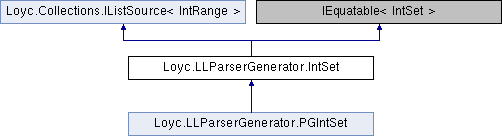Enhanced C# Language of your choice: library documentation
Loyc.LLParserGenerator.IntSet Class Reference

Represents a set of characters (e.g. 'A'..'Z' | 'a'..'z' | '_'), or a set of token IDs. More...

Source file:
Inheritance diagram for Loyc.LLParserGenerator.IntSet:## Remarks

Represents a set of characters (e.g. 'A'..'Z' | 'a'..'z' | '_'), or a set of token IDs.

This class was written for, and is used by, LLLPG. In addition to set operations like union and Subtract, one of its main features is that it can convert a set of integers or characters to/from a string form like "[A-Z]" or "(65..90)".

## Public fields

bool IsCharSet = false
Controls the default stringization mode. When IsCharSet, the set "(36, 65..90, 126)" prints as "[\$A-Z~]". IsCharSet is false by default. More...

## Public static fields

static readonly IntSet All = IntSet.Without(EmptyArray<int>.Value)

static readonly IntSet Empty = new IntSet()

static readonly Symbol S_Equivalent = GSymbol.Get("Equivalent")

static readonly Symbol S_SameRangeList = GSymbol.Get("SameRangeList")

static readonly Symbol S_Identical = GSymbol.Get("Identical")

## Properties

bool IsInverted` [get]`
When Inverted is true, the set behaves as if it contains the opposite set of items. That is, membership tests that succeeded when Inverted was false will fail, and vice versa. More...

bool IsEmptySet` [get]`
Returns true iff the set is empty. When the set is inverted and contains the set of all integers, that also counts as empty. More...

bool ContainsEverything` [get]`
Returns true iff the set covers all integers. This includes the common scenario that the set is empty but inverted. More...

long? Size` [get]`
Gets the number of integers whose membership test would succeed (the maximum possible value is 0x100000000L). More...

long SizeIgnoringInversion` [get]`

IntRange this[int index]` [get]`

int Count` [get]`

## Public Member Functions

IntSet Inverted ()

IntSet (bool isCharSet=false, bool inverted=false)

IntSet (IntRange r, bool isCharSet=false, bool inverted=false)

IntSet (bool isCharSet, bool inverted, params IntRange[] list)

bool Contains (int ch)

IntSet Union (IntSet r, bool cloneWhenOneIsEmpty=false)

IntSet Intersection (IntSet r, bool subtract=false, bool subtractThis=false)

IntSet Subtract (IntSet other)

IntSet EquivalentInverted ()
Computes the equivalent inverted set, e.g. if the set is `'b'..'y'`, the equivalent inverted set is `~(int.MinValue..'a' | 'z'..int.MaxValue)`. More...

IntSet Clone ()

override string ToString ()
Prints the character set using regex syntax, e.g. [\$a-z] means "EOF or a to z", [^
\r] means "not \n or \r". Use ToString(false) if this is an integer set. More...

string ToString (bool charSet)

IEnumerator< IntRangeGetEnumerator ()

IEnumerator< IntRangeGetEnumerator (bool obeyInversion)

IntRange TryGet (int index, out bool fail)

override bool Equals (object obj)

bool Equals (IntSet other)

override int GetHashCode ()

bool Equals (IntSet other, Symbol mode)

InternalList< IntRangeRuns ()

InternalList< IntRangeInternalRangeList ()

IEnumerable< int > IntegerSequence (bool obeyInversion)

IntSet Optimize (IntSet dontcare, bool mergeRuns=true)Public Member Functions inherited from Loyc.Collections.IListSource< IntRange >
IRange< T > Slice (int start, int count=int.MaxValue)
Returns a sub-range of this list. More...

## Static Public Member Functions

static implicit operator IntSet (int c)

static implicit operator IntSet (IntRange r)

static IntSet With (params int[] members)

static IntSet WithRanges (params int[] ranges)

static IntSet Without (params int[] members)

static IntSet WithoutRanges (params int[] ranges)

static IntSet WithChars (params int[] members)

static IntSet WithCharRanges (params int[] ranges)

static IntSet WithoutChars (params int[] members)

static IntSet WithoutCharRanges (params int[] ranges)

static IntSet Parse (string members)

static IntSet TryParse (string members)

static IntSet TryParse (string members, out int errorIndex)

## Protected Member Functions

IntSet (bool isCharSet, InternalList< IntRange > ranges, bool inverted, bool autoSimplify)

IntSet (bool isCharSet, bool inverted, bool ranges, params int[] list)

virtual IntSet New (IntSet basis, bool inverted, InternalList< IntRange > ranges)

## Protected fields

readonly InternalList< IntRange_ranges = InternalList<IntRange>.Empty
A list of non-overlapping character ranges, sorted by code point. EOF can be included in this list as character -1 (hence CharRange holds ints instead of chars). More...

## ◆ EquivalentInverted()

 IntSet Loyc.LLParserGenerator.IntSet.EquivalentInverted ( )
inline

Computes the equivalent inverted set, e.g. if the set is `'b'..'y'`, the equivalent inverted set is `~(int.MinValue..'a' | 'z'..int.MaxValue)`.

## ◆ ToString()

 override string Loyc.LLParserGenerator.IntSet.ToString ( )
inline

Prints the character set using regex syntax, e.g. [\$a-z] means "EOF or a to z", [^
\r] means "not \n or \r". Use ToString(false) if this is an integer set.

References Loyc.LLParserGenerator.IntSet.IsCharSet.

## ◆ _ranges

 readonly InternalList Loyc.LLParserGenerator.IntSet._ranges = InternalList.Empty
protected

A list of non-overlapping character ranges, sorted by code point. EOF can be included in this list as character -1 (hence CharRange holds ints instead of chars).

## ◆ IsCharSet

 bool Loyc.LLParserGenerator.IntSet.IsCharSet = false

Controls the default stringization mode. When IsCharSet, the set "(36, 65..90, 126)" prints as "[\$A-Z~]". IsCharSet is false by default.

## ◆ ContainsEverything

 bool Loyc.LLParserGenerator.IntSet.ContainsEverything
get

Returns true iff the set covers all integers. This includes the common scenario that the set is empty but inverted.

## ◆ IsEmptySet

 bool Loyc.LLParserGenerator.IntSet.IsEmptySet
get

Returns true iff the set is empty. When the set is inverted and contains the set of all integers, that also counts as empty.

## ◆ IsInverted

 bool Loyc.LLParserGenerator.IntSet.IsInverted
get

When Inverted is true, the set behaves as if it contains the opposite set of items. That is, membership tests that succeeded when Inverted was false will fail, and vice versa.

Referenced by Loyc.LLParserGenerator.IntSet.EquivalentInverted().

## ◆ Size

 long? Loyc.LLParserGenerator.IntSet.Size
get

Gets the number of integers whose membership test would succeed (the maximum possible value is 0x100000000L).